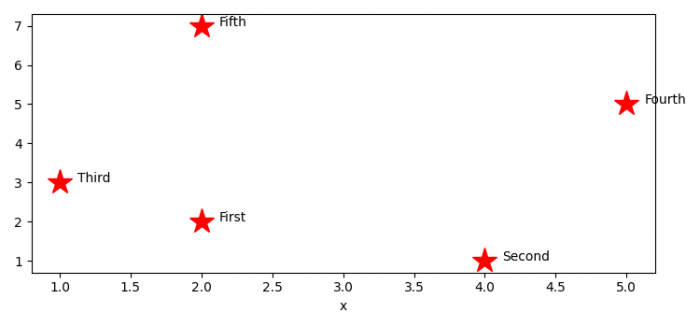# Annotating points from a Pandas Dataframe in Matplotlib plot

MatplotlibPythonData Visualization

To annotate points from a Pandas dataframe in Matplotlib, we can take the following steps −

• Set the figure size and adjust the padding between and around the subplots.

• Make a two-dimensional, size-mutable, potentially heterogeneous tabular data, with x, y and textc columns.

• Plot the columns x and y data points, using plot() method.

• Concatenate Pandas objects along a particular axis with optional set logic along the other axes.

• Iterate the Pandas object.

• Place text for each plotted points using text() method.

• To display the figure, use show() method.

## Example

import pandas as pd
import matplotlib.pyplot as plt

plt.rcParams["figure.figsize"] = [7.50, 3.50]
plt.rcParams["figure.autolayout"] = True

df = pd.DataFrame(dict(x=[2, 4, 1, 5, 2], y=[2, 1, 3, 5, 7], text=['First', 'Second', 'Third', 'Fourth', 'Fifth']))

ax = df.set_index('x')['y'].plot(style='*', color='red', ms=20)

a = pd.concat({'x': df.x, 'y': df.y, 'text': df.text}, axis=1)

for i, point in a.iterrows():
ax.text(point['x']+0.125, point['y'], str(point['text']))

plt.show()

## Output